Scripting Guide > Data Structures > Matrices > Inverse Matrices and Linear Systems
Publication date: 07/30/2020

## Inverse Matrices and Linear Systems

JMP has the following functions for computing inverse matrices: Inverse(), GInverse(), and Sweep(). The Solve() function is used for solving linear systems of equations.

### Inverse or Inv

The Inverse() function returns the matrix inverse for the square, nonsingular matrix argument. Inverse() can be abbreviated Inv. For a matrix A, the matrix product of A and Inverse(A) (often denoted A(A-1)) returns the identity matrix.

`A = [5 6, 7 8];`
`AInv = Inv( A );`
`A*A Inv;`

[1 0,0 1]

` `
`A = [1 2, 3 4];`
`AInv = Inverse( A );`
`A*AInv;`

[1 1.110223025e-16,0 1]

Note: There can be small discrepancies in the results due to floating point limitations, as illustrated in the second example.

### GInverse

The (Moore-Penrose) generalized inverse of a matrix A is any matrix G that satisfies the following conditions:

AGA=A

GAG=G

(AG)´ = AG

(GA)´ = GA

The GInverse() function accepts any matrix, including non-square ones, and uses singular-value decomposition to calculate the Moore-Penrose generalized inverse. The generalized inverse can be useful when inverting a matrix that is not full rank. Consider the following system of equations: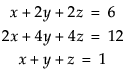Find the solution to this system using the following script:

`A = [1 2 2, 2 4 4, 1 1 1];`
`B = [6, 12, 1];`
`Show( GInverse( A ) * B );`

GInverse(A) * B = [-4, 2.5, 2.5];

### Solve

The Solve() function solves a system of linear equations. Solve() finds the vector x so that x = A-1b where A equals a square, nonsingular matrix and b is a vector. The matrix A and the vector b must have the same number of rows. Solve(A,b) is the same as Inverse(A)*b.

`A = [1 -4 2, 3 3 2, 0 4 -1];`
`b = [1, 2, 1];`
`x = Solve( A, b );`

[-16.9999999999999, 4.99999999999998, 18.9999999999999]

`A*x;`

[1, 2, 0.999999999999997]

Note: There can be small discrepancies in the results due to floating point limitations, as illustrated in the example.

### Sweep

The Sweep() function inverts parts (or pivots) of a square matrix. If you sequence through all of the pivots, you are left with the matrix inverse. Normally the matrix must be positive definite (or negative definite) so that the diagonal pivots never go to zero. Sweep() does not check whether the matrix is positive definite. If the matrix is not positive definite, then it still works, as long as no zero pivot diagonals are encountered. If zero (or near-zero) diagonal pivots are encountered on a full sweep, then the result is a g2 generalized inverse if the zero pivot row and column are zeroed.

Suppose matrix E consists of smaller matrix partitions, A, B, C, and D: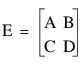The syntax for Sweep() appears as follows:

`Sweep( E, [...] );`

[...] indicates partition A.

This produces the matrix result equivalent to the following: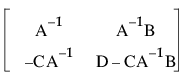Notes:

The submatrix in the A position becomes the inverse.

The submatrix in the B position becomes the solution to Ax = B.

The submatrix in the C position becomes the solution to xA = C.

#### Use of the Sweep Function

Sweep() is sequential and reversible:

A = Sweep( A, {i,j} ) is the same as A = Sweep( Sweep( A, i ), j ). It is sequential.

A = Sweep( Sweep( A, i ), i ) restores A to its original values. It is reversible.

If you have a cross-product matrix partitioned as follows: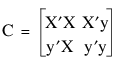Then after sweeping through the indices of XX, the result appears as follows: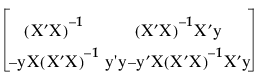The partitions are recognizable to statisticians as follows:

the least squares estimates for the model Y = Xb + e in the upper right

the sum of squared errors in the lower right

a matrix proportional to the covariance of the estimates in the upper left

The Sweep function is useful in computing the partial solutions needed for stepwise regression.

The index argument is a vector that lists the rows (or equivalently the columns) on which you want to sweep the matrix. For example, if E is a 4x4 matrix, to sweep on all four rows to get E-1 requires these commands:

`E = [ 5 4 1 1, 4 5 1 1, 1 1 4 2, 1 1 2 4];`
`Sweep( E, [1, 2, 3, 4] );`

[0.56 -0.44 -0.02 -0.02,

-0.44 0.56 -0.02 -0.02,

-0.02 -0.02 0.34 -0.16,

-0.02 -0.02 -0.16 0.34]

`Inverse( E ); // notice that these results are the same`

[0.56 -0.44 -0.02 -0.02,

-0.44 0.56 -0.02 -0.02,

-0.02 -0.02 0.34 -0.16,

-0.02 -0.02 -0.16 0.34]

Note: For a tutorial on Sweep(), and its relation to the Gauss-Jordan method of matrix inversion, see Goodnight, J.H. (1979) “A tutorial on the SWEEP operator.” The American Statistician, August 1979, Vol. 33, No. 3. pp. 149–58.

Sweep() is further demonstrated in the ANOVA Example.

### Determinant

The Det() function returns the determinant of a square matrix. The determinant of a 2 × 2 matrix is the difference of the diagonal products, as demonstrated below. Determinants for n × n matrices are defined recursively as a weighted sum of determinants for (n - 1) × (n - 1) matrices. For a matrix to have an inverse, the determinant must be nonzero.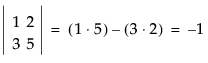`F = [1 2, 3 5];`
`Det( F );`

-1